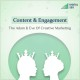Home Entity power
Uncategorized

# power

In physics, power is the rate of doing work. It is equivalent to an amount of energy consumed per unit time. In the SI system, the unit of power is the joule per second (J/s), known as the watt in honor of James Watt, the eighteenth-century developer of the steam engine. The integral of power over time defines the work performed. Because this integral depends on the trajectory of the point of application of the force and torque, this calculation of work is said to be path dependent. The same amount of work is done when carrying a load up a flight of stairs whether the person carrying it walks or runs, but more power is needed for running because the work is done in a shorter amount of time. The output power of an electric motor is the product of the torque that the motor generates and the angular velocity of its output shaft. The power involved in moving a vehicle is the product of the traction force of the wheels and the velocity of the vehicle. The rate at which a light bulb converts electrical energy into light and heat is measured in watts—the higher the wattage, the more power, or equivalently the more electrical energy is used per unit time.